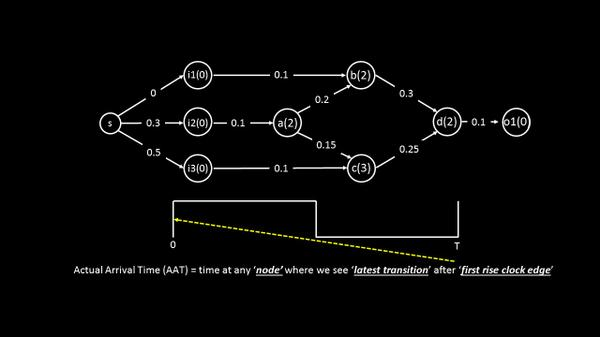# First things first – Timing Graph – Part 2

Hello

Now that we know what a timing graph is, let me unveil actual arrival time (AAT), required arrival time (RAT) and slack. We have seen these terms in a timing report, but what I will be talking about in this post is more from an algorithm viewpoint. Stay with me!!

Actual arrival time (AAT) is the time at any node … (sentence continued after below image)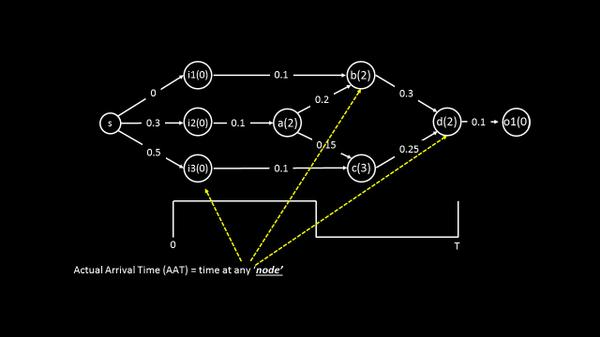… (sentence continued from above image) where we see latest transition after first rise clock edgeWith that definition, if we calculate the arrival times at nodes i1, i2, and i3, they will be 0, 0.3, and 0.5 units respectively. Let us represent actual arrival time by ‘A’ as shown below. The AAT at node ‘a’ will be AAT(i2) + wire delay which is 0.1 + the delay of node ‘a’ which is 2.

So the AAT(a) = 0.3 + 0.1 + 2 = 2.4 as shown below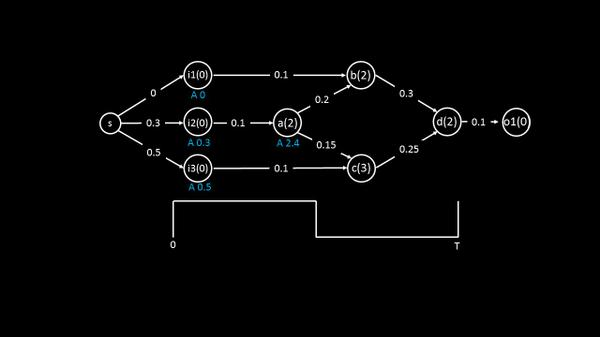The arrival time computation was still simple till this point. The trick comes when we are required to calculate the AAT at node ‘b’, as there are two incoming paths to it, one through node i1 and other through node i2, and hence two arrival times A1 and A2 as shown below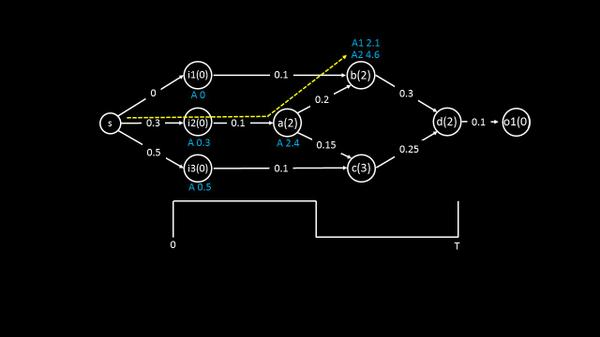The rule says we need to go for a worst case analysis for setup timing and hence, even in this case we select the worst arrival time which A2 = A = 4.6. The reason is, if in real scenario, the signal takes A2 path and if, while computation of AAT, we take A1 path (which is through i1), we might be a bit optimistic.

Same explanation goes for node ‘c’ and ‘d’ as well and is shown below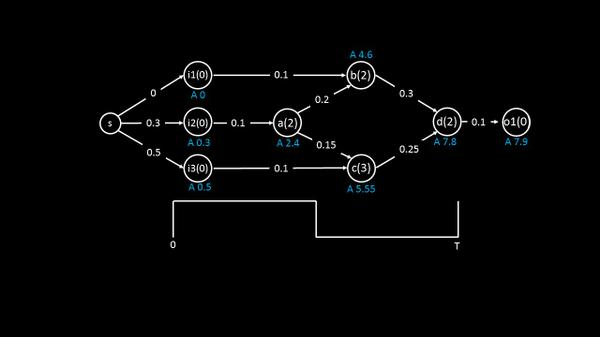Now that we have a complete picture of arrival times at every node, the next step is get the required arrival time and each node and finally the slack at each node.
Remember, I had promised in my first post, that I will make this interesting for you. Well, I think I have done it, so far. And things become exciting when I take you to next level of computation. Learning static timing analysis will be never the same and that I promise
“There comes a time when the mind takes a higher plane of knowledge but can never prove how it got there”Albert Einstein
Happy Learning!!
Posted in Uncategorized.